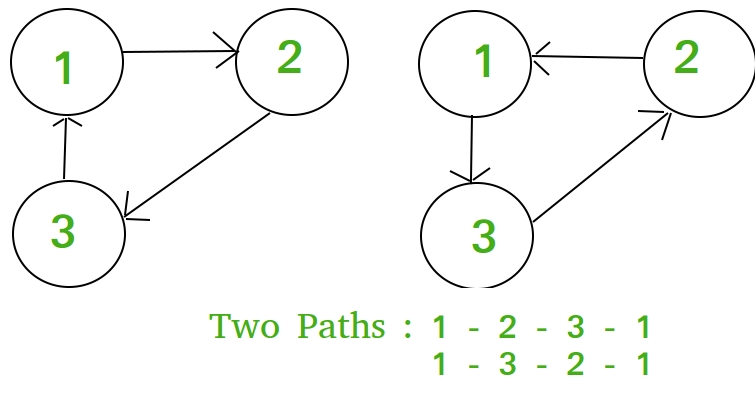# Number of loops of size k starting from a specific node

• Difficulty Level : Easy
• Last Updated : 08 Aug, 2022

Given two positive integer n, k. Consider an undirected complete connected graph of n nodes in a complete connected graph. The task is to calculate the number of ways in which one can start from any node and return to it by visiting K nodes.

Examples:

Input : n = 3, k = 3
Output : 2Input : n = 4, k = 2
Output : 3

Lets f(n, k) be a function which return number of ways in which one can start from any node and return to it by visiting K nodes.
If we start and end from one node, then we have K – 1 choices to make for the intermediate nodes since we have already chosen one node in the beginning. For each intermediate choice, you have n – 1 options. So, this will yield (n – 1)k – 1 but then we have to remove all the choices cause smaller loops, so we subtract f(n, k – 1)

So, recurrence relation becomes,
f(n, k) = (n - 1)k - 1 - f(n, k - 1) with base case f(n, 2) = n - 1.
On expanding,
f(n, k) = (n - 1)k - 1 - (n - 1)k - 2 + (n - 1)k - 3 ..... (n - 1) (say eqn 1)
Dividing f(n, k) by (n - 1),
f(n, k)/(n - 1) = (n - 1)k - 2 - (n - 1)k - 3 + (n - 1)k - 4 ..... 1 (say eqn 2)
On adding eqn 1 and eqn 2,
f(n, k) + f(n, k)/(n - 1) = (n - 1)k - 1 + (-1)k
f(n, k) * ( (n -1) + 1 )/(n - 1) = (n - 1)k - 1 + (-1)kBelow is the implementation of this approach:

## C++

 // C++ Program to find number of cycles of length// k in a graph with n nodes.#include using namespace std; // Return the Number of ways from a// node to make a loop of size K in undirected// complete connected graph of N nodesint numOfways(int n, int k){    int p = 1;     if (k % 2)        p = -1;     return (pow(n - 1, k) + p * (n - 1)) / n;} // Driven Programint main(){    int n = 4, k = 2;    cout << numOfways(n, k) << endl;    return 0;}

## Java

 // Java Program to find number of// cycles of length k in a graph// with n nodes.public class GFG {         // Return the Number of ways    // from a node to make a loop    // of size K in undirected    // complete connected graph of    // N nodes    static int numOfways(int n, int k)    {        int p = 1;             if (k % 2 != 0)            p = -1;             return (int)(Math.pow(n - 1, k)                    + p * (n - 1)) / n;    }         // Driver code    public static void main(String args[])    {        int n = 4, k = 2;             System.out.println(numOfways(n, k));    }} // This code is contributed by Sam007.

## Python3

 # python Program to find number of# cycles of length k in a graph# with n nodes. # Return the Number of ways from a# node to make a loop of size K in# undirected complete connected# graph of N nodesdef numOfways(n,k):         p = 1     if (k % 2):        p = -1     return (pow(n - 1, k) +                   p * (n - 1)) / n # Driver coden = 4k = 2print (numOfways(n, k)) # This code is contributed by Sam007.

## C#

 // C# Program to find number of cycles// of length k in a graph with n nodes.using System; class GFG {         // Return the Number of ways from    // a node to make a loop of size    // K in undirected complete    // connected graph of N nodes    static int numOfways(int n, int k)    {        int p = 1;             if (k % 2 != 0)            p = -1;             return (int)(Math.Pow(n - 1, k)                     + p * (n - 1)) / n;    }         // Driver code    static void Main()    {        int n = 4, k = 2;                 Console.Write( numOfways(n, k) );    }} // This code is contributed by Sam007.

## PHP

 

## Javascript

 

Output:

3

My Personal Notes arrow_drop_up